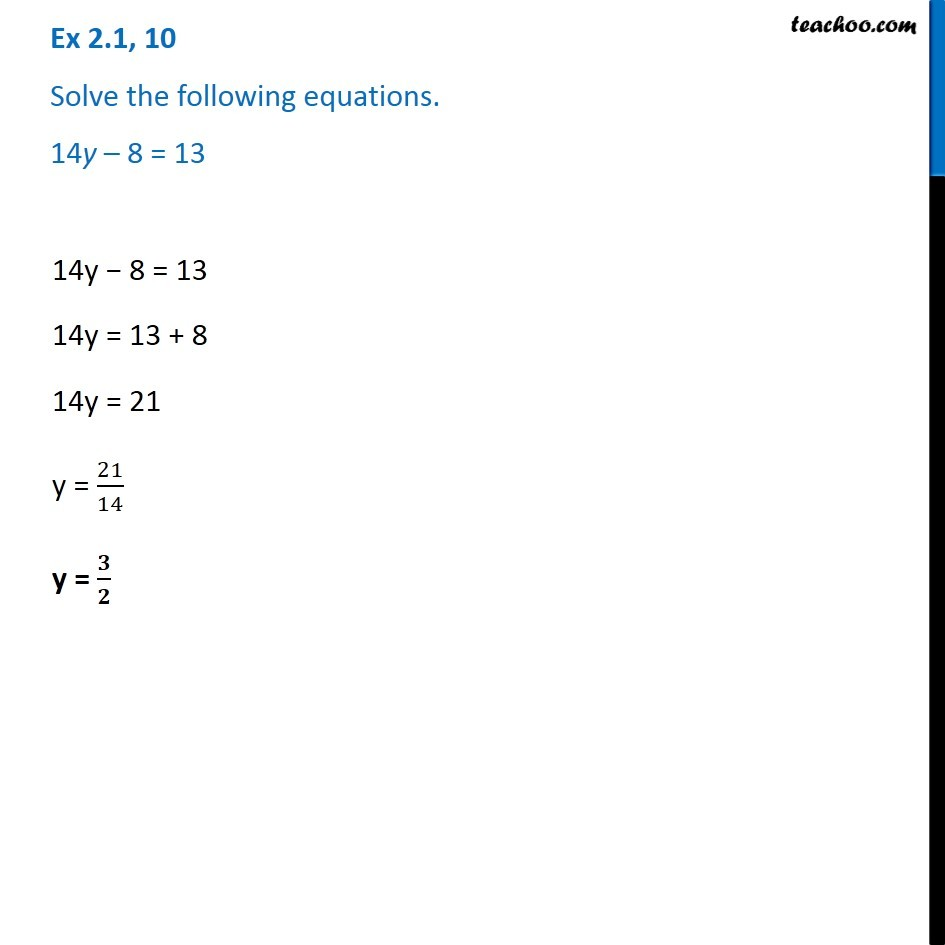Solving easy equations

Chapter 2 Class 8 Linear Equations in One Variable
Serial order wiseLearn in your speed, with individual attention - Teachoo Maths 1-on-1 Class

### Transcript

Question 10 - Chapter 2 Class 8 NCERT - Linear Equations in one variable Solve the following equations. 14y – 8 = 13 14y − 8 = 13 14y = 13 + 8 14y = 21 y = 21/14 y = 3/2